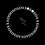# CS Problems

These are a collection of some interesting problems that I came up while reading CS. I'll be adding more.

1. Given any 2 Rubik's Cube configurations, find out the minimum distance between them (open problem).
2. Given any matrix, a legal move defined as performing a cyclic shift on a column or a row. Given two matrices with exactly the same elements, find the minimum legal moves required to obtain second matrix from the first one.
3. There are $M$ shoppers and $C$ counters at hyper mall. $k^\text{th}$ shopper takes $t_k$ time to shop and $b_k$ time to complete the billing process. Given the values $t_i$ and $b_i$, find an way to arrange the shoppers at the counters such that the last shopper leaves as early as possible.
4. You are given the rates of stocks for the next 30 days and while you can buy and sell multiple times, you can only hold at most one position at any time. Determine the maximum profit which you can make.
5. For any set $K = \{a_1, a_2, \dots, a_n\}$ of integers, $\text{SXOR}(K) = a_1 \text{ XOR } a_2 \text{ XOR } \dots \text{ XOR } a_n$. Given any set $S$, find the set $A$ such that $A \subseteq S$ and $\text{SXOR}(A)$ is maximal.

Published Problems

If you have any problem understanding the statement or require some input for a particular problem, please comment below.Note by Arulx Z
4 years, 7 months ago

This discussion board is a place to discuss our Daily Challenges and the math and science related to those challenges. Explanations are more than just a solution — they should explain the steps and thinking strategies that you used to obtain the solution. Comments should further the discussion of math and science.

When posting on Brilliant:

• Use the emojis to react to an explanation, whether you're congratulating a job well done , or just really confused .
• Ask specific questions about the challenge or the steps in somebody's explanation. Well-posed questions can add a lot to the discussion, but posting "I don't understand!" doesn't help anyone.
• Try to contribute something new to the discussion, whether it is an extension, generalization or other idea related to the challenge.

MarkdownAppears as
*italics* or _italics_ italics
**bold** or __bold__ bold
- bulleted- list
• bulleted
• list
1. numbered2. list
1. numbered
2. list
Note: you must add a full line of space before and after lists for them to show up correctly
paragraph 1paragraph 2

paragraph 1

paragraph 2

[example link](https://brilliant.org)example link
> This is a quote
This is a quote
    # I indented these lines
# 4 spaces, and now they show
# up as a code block.

print "hello world"
# I indented these lines
# 4 spaces, and now they show
# up as a code block.

print "hello world"
MathAppears as
Remember to wrap math in $$ ... $$ or $ ... $ to ensure proper formatting.
2 \times 3 $2 \times 3$
2^{34} $2^{34}$
a_{i-1} $a_{i-1}$
\frac{2}{3} $\frac{2}{3}$
\sqrt{2} $\sqrt{2}$
\sum_{i=1}^3 $\sum_{i=1}^3$
\sin \theta $\sin \theta$
\boxed{123} $\boxed{123}$